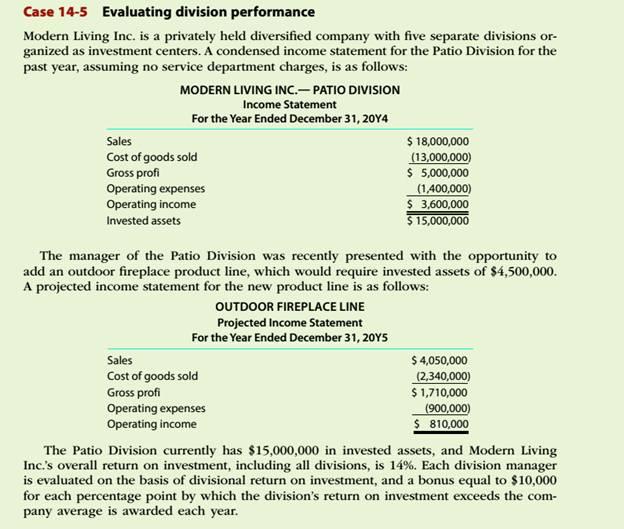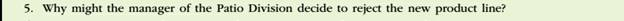Chapter
Section
Textbook ProblemTo determine

Concept Introduction:

Return on investment (ROI):

Return on investment is a profitability ratio that represents the percentage return on the investment made. It is calculated by dividing the Net Income by the Average total assets. The formulas to calculate the ROI are as follows:

ROI = Operating IncomeAverage total assets

ROI (Expanded)= Operating IncomeSales ×SalesAverage total assets

Or

ROI = Profit Margin Ratio ×Asset Turnover ratio

To Indicate:

The reason why the manager would decide to reject the new product line

Explanation

The Return on investment for the patio division for the past year is calculated as follows:

 Operating Income (A) $3,600,000 Invested Assets (B)$ 15,000,000 Return on Investment (ROI) = A/B = 24%

The estimated return on investment after adding the new product line is calculated as follows:

Still sussing out bartleby?

Check out a sample textbook solution.

See a sample solution

The Solution to Your Study Problems

Bartleby provides explanations to thousands of textbook problems written by our experts, many with advanced degrees!

Get Started

Find more solutions based on key concepts